# Homework Help forPhysics

70,505 results

Physics is the study of matter, motion, energy and force. It is a fundamental science that seeks to understand and explain the universe around us and beyond.p003 asked for the first timesreejith asked for the first timena2195413 asked for the first timekirtiriya03 asked for the first timecaesarismaeel asked for the first time

Use dimensional analysis to obtain the SI unit of k in terms of fundamental quantities in the expression below. a is the acceleration, x is the displacement, m is the mass of the oscillating object, and k is the spring constant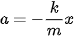nondi-chris asked for the first time

Three vectors are given by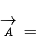3i + 3j - 3k,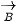= -i +4j +2k, and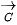= 2i + 2j + k. Find:

The component of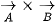alongStart filling in the gaps now